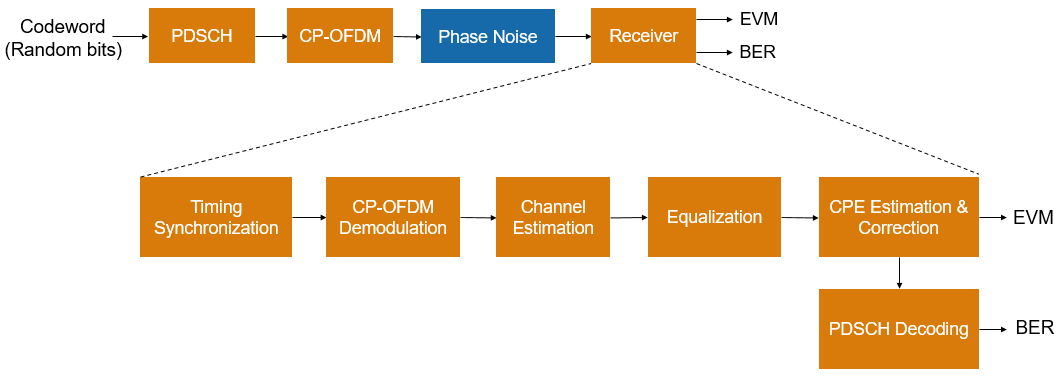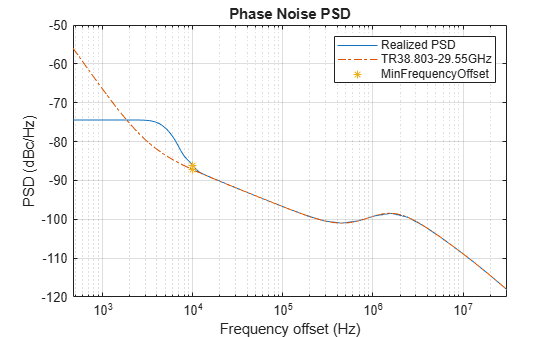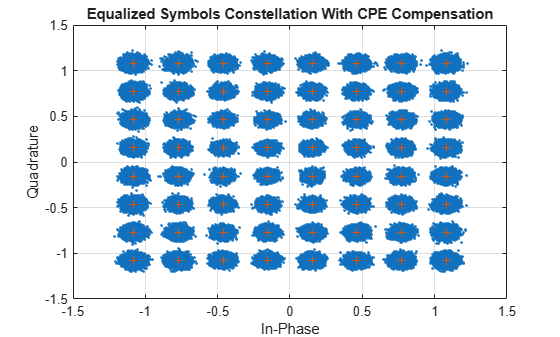# NR Phase Noise Modeling and Compensation

This example demonstrates the impact of phase noise in a 5G OFDM system and shows how to use phase tracking reference signal (PT-RS) in compensating the common phase error (CPE). The example measures error vector magnitude (EVM) and bit error rate (BER) with and without CPE compensation.

### Introduction

In 5G NR, 3GPP introduces a new reference signal, named phase tracking reference signal (PT-RS), to deal with oscillator noise. The noise incurred in the oscillators results in phase modulation of the information signal, leading to significant changes in the frequency spectrum and timing properties of the information signal. This phenomenon related to oscillators is called phase noise. Phase noise produced in local oscillators introduces a significant degradation at millimeter wave (mmWave) frequencies, depending on the power spectral density of phase noise. Phase noise leads to CPE and intercarrier interference (ICI). CPE leads to an identical rotation of a received symbol in each subcarrier. ICI leads to a loss of orthogonality between the subcarriers. Due to the distributed structure of PT-RS in frequency domain, the example primarily uses PT-RS to estimate and minimize the effect of CPE on system performance. The example applies phase noise on the waveform consisting of physical downlink shared channel (PDSCH) and shows the change in EVM and BER without and with CPE compensation. This figure shows the processing chain implemented in this example.### Phase Noise Modeling

The oscillator power spectral density models the phase noise. This example uses a multipole zero model to approximate the power spectral density of the oscillator. Use the `PNModel` field of the `simParameters` structure to select the phase noise model: 'A', 'B', or 'C'. Parameter sets 'A' and 'B' are obtained from practical oscillators operating at 30 GHz and 60 GHz, respectively, as described in TDoc R1-163984. The parameter set 'C' is obtained from the practical oscillator operating at 29.55 GHz, as described in TR 38.803 Section 6.1.10.

The example uses a carrier with a subcarrier spacing of 60 kHz for a transmission bandwidth of 50 MHz.

```% Configure carrier carrier = nrCarrierConfig; carrier.SubcarrierSpacing = 60; carrier.CyclicPrefix = 'normal'; carrier.NSizeGrid = 66; % Set the operating frequency and choose the phase noise model simParameters = []; simParameters.Fc = 30e9; % Frequency in Hz simParameters.PNModel = 'A'; % 'A' (TDoc R1-163984 Set A), 'B' (TDoc R1-163984 Set B), 'C' (TR 38.803) % Get the sample rate ofdmInfo = nrOFDMInfo(carrier); sr = ofdmInfo.SampleRate; % Phase noise level foffsetLog = (4:0.2:log10(sr/2)); % Model offset from 1e4 Hz to sr/2 Hz foffset = 10.^foffsetLog; % Linear frequency offset pn_PSD = hPhaseNoisePoleZeroModel(foffset,simParameters.Fc,simParameters.PNModel); % dBc/Hz % Set phase noise level pnoise = comm.PhaseNoise('FrequencyOffset',foffset,'Level',pn_PSD,'SampleRate',sr); pnoise.RandomStream = "mt19937ar with seed"; % Visualize spectrum mask of phase noise figure semilogx(foffset,pn_PSD) xlabel('Frequency offset (Hz)') ylabel('dBc/Hz') title('Phase noise magnitude response') grid on```### Configure PDSCH

The example configures PDSCH occupying the complete carrier with modulation scheme set to '64QAM' and number of layers set to 1. The example defaults to a single layer and a single codeword of random uncoded bits.

```% Set PDSCH parameters pdsch = nrPDSCHConfig; pdsch.PRBSet = 0:carrier.NSizeGrid-1; pdsch.SymbolAllocation = [0 14]; pdsch.Modulation = '64QAM'; pdsch.NumLayers = 1; pdsch.MappingType = 'A'; pdsch.NID = 1; pdsch.RNTI = 2; % Set DM-RS parameters pdsch.DMRS.DMRSConfigurationType = 1; pdsch.DMRS.DMRSTypeAPosition = 2; pdsch.DMRS.DMRSAdditionalPosition = 0; pdsch.DMRS.DMRSLength = 1; pdsch.DMRS.DMRSPortSet = []; pdsch.DMRS.NumCDMGroupsWithoutData = 1; pdsch.DMRS.NIDNSCID = 1; pdsch.DMRS.NSCID = 0; % Set PT-RS parameters pdsch.EnablePTRS = 1; pdsch.PTRS.TimeDensity = 1; pdsch.PTRS.FrequencyDensity = 2; pdsch.PTRS.REOffset = '00'; pdsch.PTRS.PTRSPortSet = [];```

### Generate Waveform

The waveform is generated for 2 frames and the field `NumFrames` of `simParameters` structure controls the number of frames of the waveform. The steps involved are:

• Generate random codeword with the bit capacity of PDSCH

• Get the PDSCH symbols for the random codeword and map them to grid

• Generate and map DM-RS symbols to grid

• Generate and map PT-RS symbols to grid

• Perform OFDM modulation for the complete grid of all frames

```% Number of frames to generate the waveform simParameters.NumFrames = 2; % Get the number of slots in the waveform and number of symbols in a slot numSlots = carrier.SlotsPerFrame*simParameters.NumFrames; nSlotSymb = carrier.SymbolsPerSlot; % Initialize the grid for specified number of frames txGrid = zeros(carrier.NSizeGrid*12,nSlotSymb*numSlots,pdsch.NumLayers); % Processing loop txbits = []; rng('default') for slotIdx = 0:numSlots - 1 % Set slot number carrier.NSlot = slotIdx; % Get PDSCH indices and structural information [pdschInd,pdschIndicesInfo] = nrPDSCHIndices(carrier,pdsch); % Generate random codeword(s) numCW = pdsch.NumCodewords; % Number of codewords data = cell(1,numCW); for i = 1:numCW data{i} = randi([0 1],pdschIndicesInfo.G(i),1); txbits = [txbits; data{i}]; %#ok<AGROW> end % Get modulated symbols pdschSym = nrPDSCH(carrier,pdsch,data); % Get DM-RS symbols and indices dmrsSym = nrPDSCHDMRS(carrier,pdsch); dmrsInd = nrPDSCHDMRSIndices(carrier,pdsch); % Get PT-RS symbols and indices ptrsSym = nrPDSCHPTRS(carrier,pdsch); ptrsInd = nrPDSCHPTRSIndices(carrier,pdsch); % Resource element mapping to slot grid slotGrid = nrResourceGrid(carrier,pdsch.NumLayers); slotGrid(pdschInd) = pdschSym; slotGrid(dmrsInd) = dmrsSym; slotGrid(ptrsInd) = ptrsSym; % Generate txGrid for all frames by mapping slotGrid at respective % locations txGrid(:,slotIdx*nSlotSymb+1:(slotIdx+1)*(nSlotSymb),:) = slotGrid; end % Perform OFDM modulation carrier.NSlot = 0; % Reset the slot number to 0 for OFDM modulation txWaveform = nrOFDMModulate(carrier,txGrid);```

### Apply Phase Noise

Apply phase noise to the transmitted waveform. To clearly observe the impact of phase noise, the example does not apply any thermal noise or channel model in addition to phase noise. The example applies the same phase noise to all the layers.

`rxWaveform = pnoise(txWaveform);`

Before returning the equalized PDSCH symbols and decoded bits, the receiver performs these steps:

• Timing synchronization

• OFDM demodulation

• Channel estimation

• Equalization

• CPE estimation and correction

• PDSCH decoding

For the CPE estimation and correction step, the receiver uses the logical field `CompensateCPE` of the `simParameters` structure. Because the example does not use a propagation channel, the steps of timing synchronization, channel estimation, and equalization are not strictly necessary. However, these steps help investigate the phase noise effects if you introduce a channel.

The example shows the equalized constellation symbols, EVM, and bit error rate, with and without CPE compensation.

#### Case 1: Without CPE Compensation

To disable the CPE compensation, set the field `CompensateCPE` of `simParameters` structure to 0.

```simParameters.CompensateCPE = 0; [eqSymbols,rxbits] = practicalReceiver(carrier,pdsch,simParameters,rxWaveform); refSymbols = getConstellationPoints(pdsch); % Display the constellation diagram figure plot(eqSymbols,'.') hold on plot(refSymbols,'+') title('Equalized Symbols Constellation Without CPE Compensation') grid on xlabel('In-Phase') ylabel('Quadrature')``````% Display RMS EVM evm = comm.EVM('ReferenceSignalSource','Estimated from reference constellation','ReferenceConstellation',refSymbols); fprintf('RMS EVM (in percent) for equalized symbols without CPE compensation: %f%% \n',evm(eqSymbols))```
```RMS EVM (in percent) for equalized symbols without CPE compensation: 7.431048% ```
```% Display bit error rate errorRate = nnz(rxbits-txbits)/numel(txbits); fprintf('Bit error rate without CPE compensation: %f \n',errorRate)```
```Bit error rate without CPE compensation: 0.005525 ```

#### Case 2: With CPE Compensation

To enable the CPE compensation, set the field `CompensateCPE` of `simParameters` structure to 0. Use PT-RS to estimate the CPE at all the OFDM symbol locations in a slot. Correct the CPE in the OFDM symbol locations within the range of PT-RS OFDM symbols.

```simParameters.CompensateCPE = 1; [eqSymbolsCPE,rxbitsCPE] = practicalReceiver(carrier,pdsch,simParameters,rxWaveform); % Display the constellation diagram figure plot(eqSymbolsCPE,'.') hold on plot(refSymbols,'+') title('Equalized Symbols Constellation With CPE Compensation') grid on xlabel('In-Phase') ylabel('Quadrature')``````% Display RMS EVM fprintf('RMS EVM (in percent) for equalized symbols with CPE compensation: %f%% \n',evm(eqSymbolsCPE))```
```RMS EVM (in percent) for equalized symbols with CPE compensation: 4.557690% ```
```% Display bit error rate errorRateCPE = nnz(rxbitsCPE-txbits)/numel(txbits); fprintf('Bit error rate with CPE compensation: %f \n',errorRateCPE)```
```Bit error rate with CPE compensation: 0.000052 ```

### Further Exploration

• To visualize the impact of phase noise, change the carrier frequency, subcarrier spacing, number of resource blocks, modulation scheme, and the number of frames.

• To see the effects of phase noise on the constellation, change the phase noise model.

• To analyze the effect of CPE compensation with different configurations, change the time and frequency density of PT-RS.

• Visualize the impacts of phase noise by including thermal noise and channel models.

### Summary

This example demonstrates the impact of phase noise and shows how to estimate and correct CPE with PT-RS. The example also shows that CPE compensation reduces the EVM and improves bit error rate. The displayed constellation plot shows huge ICI in mmWave frequencies, indicating that ICI compensation needs to be performed in addition to CPE compensation.

### Local Functions

```function [eqSymbols,rxbits] = practicalReceiver(carrier,pdsch,params,rxWaveform) % Returns equalized modulated symbols after performing the timing % estimation, OFDM demodulation, channel estimation, MMSE equalization, % CPE estimation and correction, and PDSCH decoding. % Get the current slot number, number of slots, number of symbols % per slot, and total number of symbols nSlot = carrier.NSlot; numSlots = carrier.SlotsPerFrame*params.NumFrames; nSlotSymb = carrier.SymbolsPerSlot; numTotalSymbols = numSlots*nSlotSymb; % Get reference grid with DM-RS symbols dmrsSymCell = cell(1,numSlots); dmrsIndCell = cell(1,numSlots); refGrid = zeros(carrier.NSizeGrid*12,numTotalSymbols,pdsch.NumLayers); for NSlot = 0:numSlots-1 carrier.NSlot = NSlot; slotGrid = nrResourceGrid(carrier,pdsch.NumLayers); dmrsSymCell{NSlot+1} = nrPDSCHDMRS(carrier,pdsch); dmrsIndCell{NSlot+1} = nrPDSCHDMRSIndices(carrier,pdsch); slotGrid(dmrsIndCell{NSlot+1}) = dmrsSymCell{NSlot+1}; refGrid(:,NSlot*nSlotSymb+1:(NSlot+1)*(nSlotSymb),:) = slotGrid; end % Perform timing estimation and correction carrier.NSlot = nSlot; offset = nrTimingEstimate(carrier,rxWaveform,refGrid); waveformSync = rxWaveform(1+offset:end,:); % Perform OFDM demodulation on the received data to recreate the % resource grid, including padding in the event that practical % synchronization results in an incomplete slots being demodulated rxGrid = nrOFDMDemodulate(carrier,waveformSync); [K,L,R] = size(rxGrid); if (L < numTotalSymbols) rxGrid = cat(2,rxGrid,zeros(K,numTotalSymbols-L,R)); end % Declare storage variables eqSymbols = []; % equalized symbols for constellation plot rxbits = []; for NSlot = 0:numSlots-1 % Extract grid for current slot currentGrid = rxGrid(:,NSlot*nSlotSymb+(1:nSlotSymb),:); % Get the PDSCH resources carrier.NSlot = NSlot; dmrsSymbols = dmrsSymCell{NSlot+1}; dmrsIndices = dmrsIndCell{NSlot+1}; ptrsSymbols = nrPDSCHPTRS(carrier,pdsch); ptrsIndices = nrPDSCHPTRSIndices(carrier,pdsch); [pdschIndices,pdschIndicesInfo] = nrPDSCHIndices(carrier,pdsch); % Channel estimation [estChannelGrid,noiseEst] = nrChannelEstimate(currentGrid,dmrsIndices,dmrsSymbols,"CDMLengths",pdsch.DMRS.CDMLengths); % Get PDSCH resource elements from the received grid [pdschRx,pdschHest] = nrExtractResources(pdschIndices,currentGrid,estChannelGrid); % Equalization pdschEq = nrEqualizeMMSE(pdschRx,pdschHest,noiseEst); % Common phase error (CPE) estimation and correction if params.CompensateCPE % Initialize temporary grid to store equalized symbols tempGrid = nrResourceGrid(carrier,pdsch.NumLayers); % Extract PT-RS symbols from received grid and estimated % channel grid [ptrsRx,ptrsHest,~,~,~,ptrsLayerIndices] = nrExtractResources(ptrsIndices,currentGrid,estChannelGrid,tempGrid); % Equalize PT-RS symbols and map them to tempGrid ptrsEq = nrEqualizeMMSE(ptrsRx,ptrsHest,noiseEst); tempGrid(ptrsLayerIndices) = ptrsEq; % Estimate the residual channel at the PT-RS locations in % tempGrid cpe = nrChannelEstimate(tempGrid,ptrsIndices,ptrsSymbols); % Sum estimates across subcarriers, receive antennas, and % layers. Then, get the CPE by taking the angle of the % resultant sum cpe = angle(sum(cpe,[1 3 4])); % Map the equalized PDSCH symbols to tempGrid tempGrid(pdschIndices) = pdschEq; % Correct CPE in each OFDM symbol within the range of reference % PT-RS OFDM symbols if numel(pdschIndicesInfo.PTRSSymbolSet) > 0 symLoc = pdschIndicesInfo.PTRSSymbolSet(1)+1:pdschIndicesInfo.PTRSSymbolSet(end)+1; tempGrid(:,symLoc,:) = tempGrid(:,symLoc,:).*exp(-1i*cpe(symLoc)); end % Extract PDSCH symbols pdschEq = tempGrid(pdschIndices); end % Store the equalized symbols and output them for all the slots eqSymbols = [eqSymbols; pdschEq]; %#ok<AGROW> % Decode the PDSCH symbols and get the hard bits eqbits = nrPDSCHDecode(carrier,pdsch,pdschEq); for i = 1:numel(eqbits) rxbits = [rxbits; double(eqbits{i}<0)]; %#ok<AGROW> end end end function sym = getConstellationPoints(pdsch) %getConstellationPoints Constellation points % SYM = getConstellationPoints(PDSCH) returns the constellation points % SYM based on modulation schemes provided in PDSCH configuration object. sym = []; modulation = string(pdsch.Modulation); % Convert modulation scheme to string type ncw = pdsch.NumCodewords; % Number of codewords if ncw == 2 && (numel(modulation) == 1) modulation(end+1) = modulation(1); end % Get the constellation points for cwIndex = 1:ncw qm = strcmpi(modulation(cwIndex),{'QPSK','16QAM','64QAM','256QAM'})*[2 4 6 8]'; sym = [sym; nrSymbolModulate(int2bit((0:2^qm-1)',qm),modulation(cwIndex))]; %#ok<AGROW> end end```